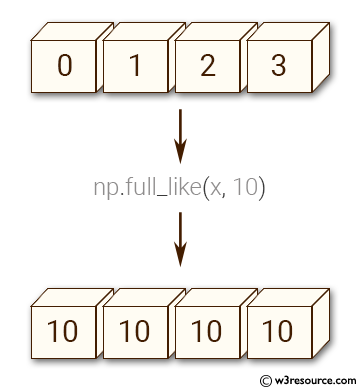﻿ NumPy: Create an array of 10's with the same shape and type of a given array - w3resource# NumPy: Create an array of 10's with the same shape and type of a given array

## NumPy: Array Object Exercise-41 with Solution

Write a NumPy program to create an array of 10's with the same shape and type of a given array.
Sample array: x = np.arange(4, dtype=np.int64).

Pictorial Presentation:Sample Solution:-

Python Code:

``````import numpy as np
x = np.arange(4, dtype=np.int64)
y = np.full_like(x, 10)
print(y)
```
```

Sample Output:

```[10 10 10 10]
```

Python Code Editor:

Have another way to solve this solution? Contribute your code (and comments) through Disqus.

What is the difficulty level of this exercise?

Test your Python skills with w3resource's quiz

﻿

## Python: Tips of the Day

Set comprehension:

```>>> m = {x ** 2 for x in range(5)}
>>> m
{0, 1, 4, 9, 16}
```